### Day 46 - Optimization - 03.18.15

Update
• Unit 3 Test on 3/27!
• If you are leaving for spring break early, please make arrangements with me to take the test before break!

Questions

Bell Ringer
1. Optimization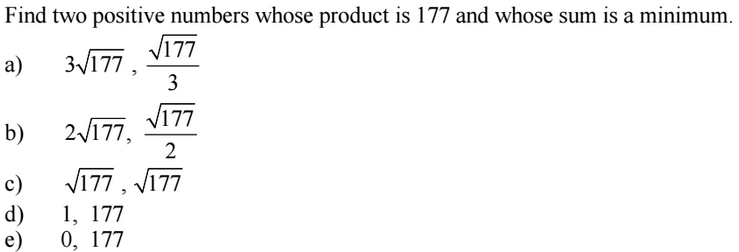1. Optimization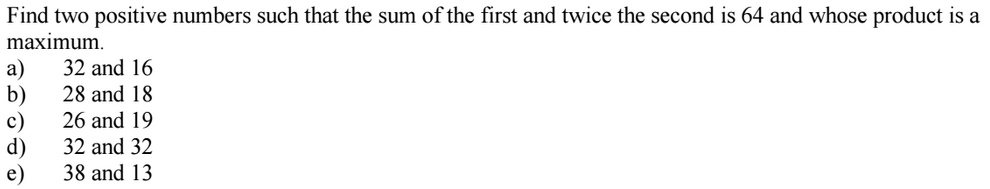1. Optimization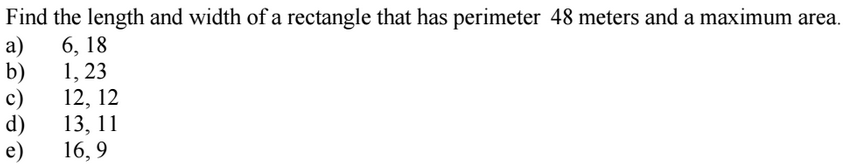1. Optimization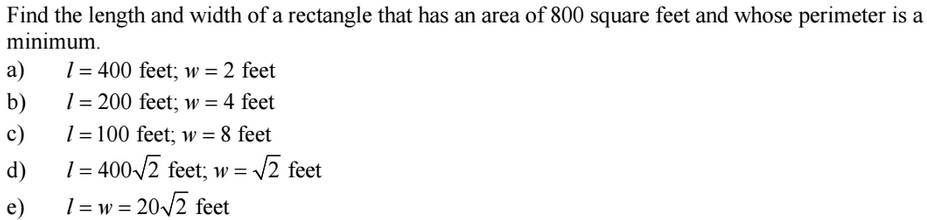1. Optimization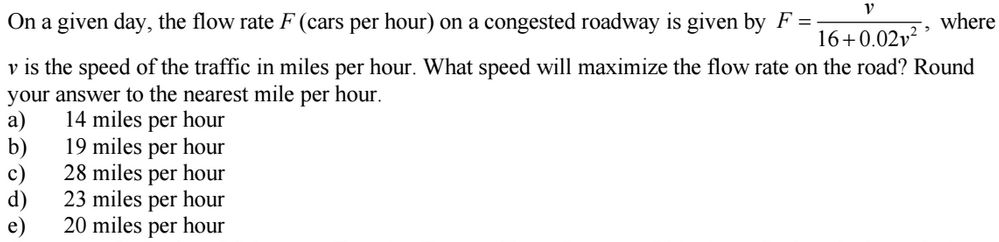Review
• Extrema/Critical Numbers (video)
• How is the derivative used to locate the minimum and maximum values of a function on a closed interval?
• Find critical numbers using differentiation.
• Find extrema on a closed interval using differentiation.
• Rolle's Theorem (videoand Mean Value Theorem  (video)
• How are Rolle's Theorem and Mean Value Theorem related to differentiation?
• Apply understanding of Rolle’s Theorem and the Mean Value Theorem.
• Increasing/Decreasing Functions (video)
• How can the first derivative to determine whether a function is increasing or decreasing?
1. Determine intervals on which a function is increasing or decreasing.
2. Apply the First Derivative Test to find relative extrema of a function.
• Concavity and Inflection Points
• How can the second derivative be used to determine the concavity of intervals of a function? (video)
1. Determine intervals on which a function in concave upward or downward.
2. Apply the Second Derivative Test to find inflection points of a function.
1. Limits at Infinity
• How can limits be used to find horizontal asymptotes of the graph of a function? (video)
1. Determine horizontal asymptotes using limits.
2. Determine finite limits at infinity.
3. Determine infinite limits at infinity.

Lesson

Exit Ticket
• Posted on the board at the end of the block
Lesson Objective(s)
• How can differentiation be used to find optimal conditions of a scenario?
Skills
1. Determine the conditions that optimize a situation.

#### In-Class Help Requests

Standard(s)
• APC.4
• Investigate asymptotic and unbounded behavior in functions.
• Includes:
• describing and understanding asymptotes in terms of graphical behavior and limits involving infinity
• APC.8
• Apply the derivative to solve problems.
• Includes:
• ​analysis of curves and the ideas of concavity and monotonicity
• optimization involving global and local extrema

Past Checkpoints